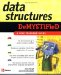# Queues Using An Array in Java

A queue is implemented in Java similar to the way you implement it in C++ except for a few tweaks, which we ll talk about in this section. The code example in this section is a queue using an array in a Java application. Two classes are defined in the example: the Queue class and the Java application class queueArrayDemo() .

The Queue class defines the same set of attributes as is defined in the C++ version of this program. However, instead of declaring an integer pointer, the Java version of this program declares a reference to an array of integers, which is called values .

The Queue class defines seven member methods including two constructors. Most of the member methods are the same as the member functions in the C++ program. However, there are a few differences in the Java Queue class: it defines two constructors, which are the first couple of member methods in the class definition.

The first version of the constructor is Queue() . The Queue() constructor is called if the instance of the Queue() class is declared without the size of the queue passed as a parameter to the constructor. The Queue() constructor uses the default size for the queue. As you ll remember from your Java programming class, the this(DEFAULT_SIZE) statement calls the Queue(int size) constructor and passes it the default size of the queue. Java does not support default parameter values like C++, so this is one way to achieve the same result.

The second version of the constructor is Queue(int size) . The Queue(int size) constructor is called whenever the size of the queue is passed as a parameter to the constructor. The size is then assigned to the size attribute of the Queue class and sets the size of the array. The array is created using the new operator, and reference to the location of the array in memory is assigned to the values attribute of the Queue class. The Queue(int size) constructor also initializes the front and back attributes.

The remaining member methods defined in the Queue class definition are the same as those defined in the Queue class definition in the C++ version of this program.

Beneath the Queue class definition is the Java application class definition called queueArrayDemo . This class defines the main() method of the application that contains nearly identical statements as those found in the main() function of the C++ program.

The first statement creates an instance of the Queue class that contains an array of 8 elements. The next three statements call the method to place values 10, 20, and 30 in the queue, respectively. Within the for loop, the dequeue() method is called to remove each item from the queue. These items are then displayed on the screen.

` class Queue {  private static final int DEFAULT_SIZE = 8;  private int size;  private int front;  private int back;  private int[] values;  public Queue()  {  this(DEFAULT_SIZE);  }  public Queue(int size)  {  this.size = size;  values = new int[size];  front = 0;  back = 0;  }  public boolean isFull()  {  if((back+1) % size == front)  {  return true;  }  else  {  return false;  }  }  public boolean isEmpty()  {  if(back == front)  {  return true;  }  else  {  return false;  }  }  public void enqueue(int x)  {  if(!isFull())  {  back = (back+1) % size;  values[back] = x;  }  }  public int dequeue()  {  if(!isEmpty())  {  front = (front+1) % size;  return values[front];  }  return 0;  } } public class queueArrayDemo{  public static void main(String args[]){  Queue queue = new Queue(8);  queue.enqueue(10);  queue.enqueue(20);  queue.enqueue(30);  for(int i=0; i<3; i++)  {  System.out.println(queue.dequeue());  }  } } `Data Structures Demystified (Demystified)
ISBN: 0072253592
EAN: 2147483647
Year: 2006
Pages: 90

Similar book on Amazon